Programming model

The WT* programming model consists of a number of threads. The program is started with one thread, and other threads can be dynamically created and terminated, forming a tree-like structure of parent-child threads. All threads operate synchronously: they share a common code with a single PC (program counter) register. At each instant some group of threads is active and they perform the current operation. Each thread can have its own local variables, and can also access all variables of its ancestors. The complexity is measured in terms of time (the number of consecutive steps) and work (the overall number of instructions performed by all active threads). The WT* framework provides a simple imperative strongly typed language, with a C-like syntax.

Program is a sequence of type definitions, variable definitions, function definitions, and statements;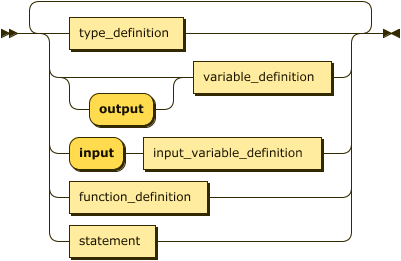Types

There are four basic types: `int`, `float`, `char` (the support for `char` is not yet ready), and `void`. User defined types (a.k.a. structs, or records) can be created with the `type` keyword by combining basic and user defined types: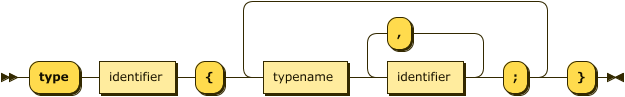so e.g. this is a valid code:
``````type point {
float x,y;
}

type my_type {
point p;
int tag;
}
``````

Variables and arrays

Variables are declared by specifying the type: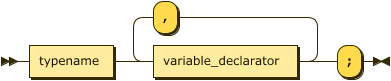The variable declarator is in the simplest form the name of the variable, e.g. `my_type quak;`. With several active threads, the variable declaration creates a separate copy of the variable in each active thread. Members of he type are accessed via the dot-notation `a.p.x`.

The language supports multidimensional arrays. The number of dimensions is fixed. The size of each dimension must be specified in the declaration. So, e.g. `int B[n,n*n];` declares a 2-dimensional array `B`. For arrays, the keyword `dim` gives the number of dimensions, e.g.

``int A[10,20,30], z = A.dim;``
results in `z == 3`. The size of the array in a given dimension can be obtained by `A.size(d)`; `A.size` is equivalent to `A.size(0)`.

Finally, a scalar variable (assignment to arrays is not supported) that is not designated as input, can be assigned to during the declaration. The full variable declarator syntax is as follows: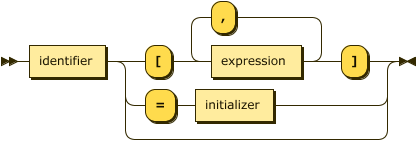The initializer can be any expression of compatible type (conversions among `int` and `float` are performed). Compound types use the bracket format, so e.g.,
``````type point {
float x,y;
}

type goo {
point p;
int a;
}

goo gle = { {3.14, -9.6} , 47 };

type ipoint {
int a,b;
}

ipoint p = gle.p;
``````
results in `p == {3, -9}`.

Input and output

The input and output is handled by input/output variables. Any variable definition can be preceded by the keyword `output` which means the variable is meant to be part of the output. The runtime environment is responsible for displaying the output variables.

Similarly, the input of data is handled by prefixing a variable definition by a keyword `input` (at most one of the `input` `output` keywords may be present). Again, the runtime environment is responsible for reading the data and initializing the variables. For obvious reasons, input variables don't support initializers.

A caveat with input arrays is that the size of the array is not known in compile time (only the number of dimensions is). Instead of the size expression, the input arrays use the don't care symbol (`_`) in definition. Following is a simple program that reads a 1-dimensional array, and returns its size:

``````input int A[_];
output int x = A.size;
``````
The format of input/output variables used by both the cli tools, and the web frontend is as follows: the static variables of simple types are the respective literals, the variables of compound types are represented as a flattened list of members enclosed in braces, e.g. `output goo gle = { {3.14, -9.6} , 47 }` would produce `{ 3.140000 -9.600000 47 }`. Arrays are row-wise bracketed lists of elements, e.g. the following program
``````input int A[_,_];
int n=A.size(0),m=A.size(1);
``````
on input `[ [ 1 2 3 ] [ 1 2 3 ] ]` has `n==2` and `m==3`. Whitespace may be added arbitrarily.

Assignment

Like initializers, assignment is supported only for scalar variables. Unlike initializers, type checking is more strict: the conversion between `float` and `int` is not performed in compound types. E.g. consider

``````type t1 {int x,y;}
type t2 {float a,b;}

t1 var1 = {4,5};
t2 var2 = {0.1,0.4};
``````
Then `t1 var3 = var2;` is okay, but `var1 = var2` is not; you need to cast the value explicitly with `var1 = (t1)var2`. Also when assigning literals of compound types, these must be typecasted, e.g. `var1 = {9,10};` is wrong, it should be `var1 = (t1){9,10}`.

As expected, assignment is left associative, so `int z = x = y = 4` works.

Expressions

Apart from assignment, which is also an expression, there are the usual operators which act only on numeric types. The binary operators are

 combined assignment `+=` `-=` `*=` `/=` `%=` `%=` works only on integers relational operators `==` `<=` `>=` `!=` `<` `>` logical operators `||` `&&` work on integers only numerical operatos `+` `-` `*` `/` `%` `^` `^` is exponentiation bitwise operators `|` `&` `~` `~` is XOR, priority is equal to multiplication
Unary increment and decrement `++` `--` are supported both as prefix and as suffix. Unary `-` and logical `!` are present. Unary postfix operator `~|` works on integers an gives the least significant bit that is set, i.e. `12~|==2`. Note that logical operators are evaluated in full, i.e. `0 && <bad stuff>` produces error.

Statements

Most of the statements have the usual semantics. The only statement not found in sequential languages is the `pardo` statement. `pardo(<var>:<range>) <statement&gy;` creates `<range>` new threads numbered `0...<range>-1`. The number is stored in a local variable `<var>`, and all threads continue to execute `<statement>`. After finishing, the threads are joined.

The SIMD mode of operation gives also a special semantics to the conditional statement: the `if (<condition>) <statement1>; else <statement2>;` construct splits the currently active threads into two groups based on the condition. The first group becomes active, and executes `<statement1>`, then the second group becomes active, and executes `<statement2>`. So the following code:

``````pardo (i:2)
if (i==0) do_someting(42);
else do_something(47);
``````
executes the two calls sequentially, whereas
`````` pardo(i:2) {
int p;
if (i==0) p=42; else p=47;
do_something(p);
}
``````
executes them in parallel.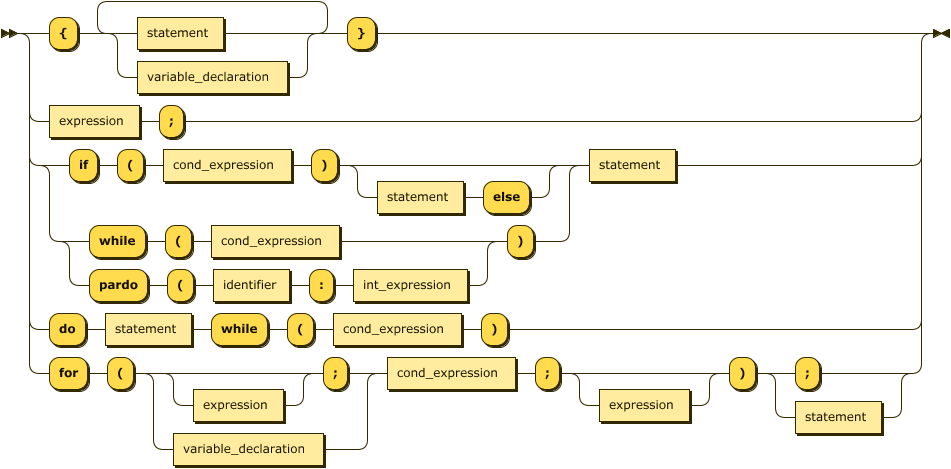Functions

The language supports C-like functions. The return type must be static (i.e. returning arrays is not allowed). Parameters are passed by value, with the caveat that the 'value' of an array is the value of the control block (basically, a pointer to the allocated memory). When passing an array as parameter, the same syntax as with input arrays is used, e.g. instead of sizes, the don't care symbol is used as a placeholder. E.g. a function that returns the length of an array would be

``````int length ( int A[_] ) {
return A.size;
}
``````
It is also possible to have separate definition and declaration (e.g. for mutual recursion). The full syntax of function definitions is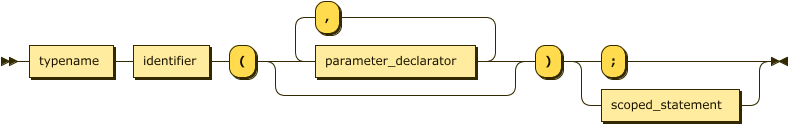where the `parameter_declarator` is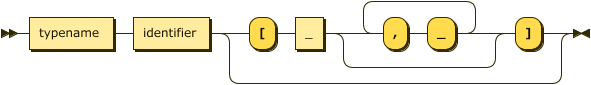The `return` (for a function returning `void`) or `return <statement>` statement is used to return from the function. There are two points concerning returning values: first, if a `return` is issued from a `pardo` statement, there would be no way to join the threads. Hence, `return` cannot appear within a pardo statement in a function body. Calling a function from a `pardo` statement, or having (possibly nested) `pardo` statements in a function is perfectly fine, though. The other point is that if the control flow reaches the end of a function (other than returning `void`) without proper `return` statement, it will end up in having the stack filled with some default values, which you probably (surely) don't want.

There are some built-in functions:
 name parameter output type value `sqrt(x)` `int` `int` `ceiling(sqrt(x))` `sqrtf(x)` `float` `float` `sqrt(x)` `log(x)` `int` `int` `ceiling(log2(x))` `logf(x)` `float` `float` `ceiling(log2(x))`
Also, technically not a function, there is a way to sort an array (incurring `log n` time and `n log n` work, where `n` is the size of the array) using a function-like syntax `sort(<array>,<key_specifier>)` where `key_specifier` refers to the type member that serves as key, so e.g.

``````type point {float x,y;}
type pair {point key;int val;}

input pair A[_];
output pair B[A.size];

pardo(i:A.size)B[i]=A[i];
sort(B,pair.key.x);
``````
on an input `[ {8 1 4} {3 4 6} {9 6 10} {1 7 5} ]` produces ```[{ 1.000000 7.000000 5 } { 3.000000 4.000000 6 } { 8.000000 1.000000 4 } { 9.000000 6.000000 10 }]```

Directives

One can use `#mode <mode>` to switch memory mode to one of `EREW`, `CREW` (default), `cCRCW`.

A file can be included (i.e. directly inserted) by `#include "<filename>"`. The filename is considered relative to the file from which the `#include` directive is used. (The include support is so far only in the cli tools).

A version `#include once "<filename>"` includes the file only if it has not been included yet. Imagine a file `filefoo` with a definition of function `foo`, and another file `filegoo` that defines function `goo` that uses `foo`, so `filegoo` contains `#include "filefoo"`. Now a file `main` that uses both `foo` and `goo` cannot contain

``````#include "filegoo"
#include "filefoo"
``````
since function `foo` would be redefined. Calling
``````#include "filegoo"
#include once "filefoo"
``````
solves the problem.

Debugging

For debugging, one can use statement `@<tag>(<condition>)`. When running in the standard mode (in `wtrun`, or using the `Run` command in the web IDE), the condition is evaluated and discarded. When running in `wtdb` or the `Debug` command, if the condition is evaluated to `true` in at least one thread, the execution is stopped. Some rudimentary facilities are provided to view the values of variables in various threads, and to continue the execution.